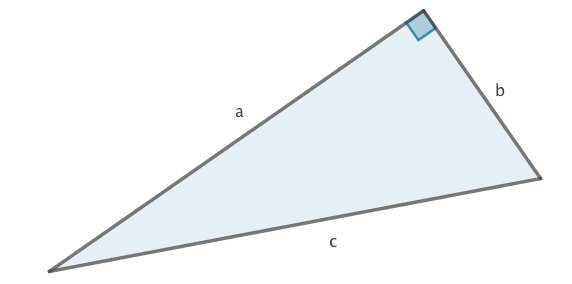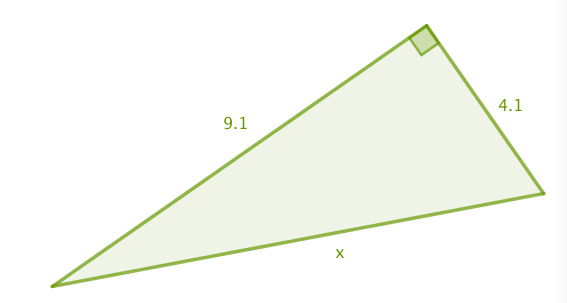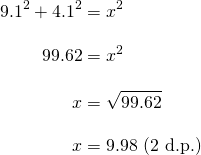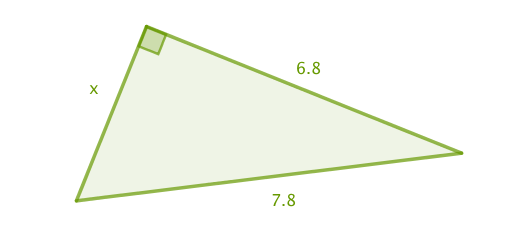# Pythagoras’ Theorem

Pythagoras’ Theorem tells us that the sides of a right angled triangle are related in the following way:If we begin by labelling our triangle with lettersandas on the following triangle,we haveThis is a beautifully simple theorem, however it is not obvious. It is named after Pythagoras, not because he discovered it but because he was probably the first to prove it.

Here is a visual, showing a square with side length, a square with side lengthand a square with side length.

Without using any algebra, this illustrates whymust be equal to. Again, not obvious to begin with, so keep looking to see why.

One difficulty with using letters in any formula is that the letters in a formula and the letters in a problem might not match up. For example, the formula above assumes that we label the hypotenuse, but in any problem the hypotenuse might be calledororor fence or anything else for that matter. That is why it is important to remember that it is the shorter sides squared, then added, that give the hypotenuse squared.

Here are two examples of working this formula:

Example 1 The longest side (opposite the right angle) is not knownIntuitively: To find the longest length, add the two shorter lengths (squared).Example 2 A shorter side is not knownIntuitively: To find a shorter length, start with the longest length (squared) and take the other shorter length (squared).# Calculating the third side on a Right Angled Triangle

All of the above from Mathisfun and Khan Academy